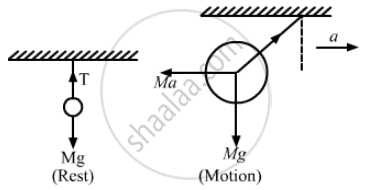Department of Pre-University Education, KarnatakaPUC Karnataka Science Class 11
Advertisement Remove all ads

# A heavy ball is suspended from the ceiling of a motor car through a light string. A transverse pulse travels at a speed of 60 cm s −1 on the string when the car is at rest - Physics

Sum

A heavy ball is suspended from the ceiling of a motor car through a light string. A transverse pulse travels at a speed of 60 cm s −1 on the string when the car is at rest and 62 cm s−1 when the car accelerates on a horizontal road. Find the acceleration of the car. Take g = 10 m s−2

Advertisement Remove all ads

#### SolutionGiven,
Speed of the transverse pulse when the car is at rest, v1 = 60 cm s−1
Speed of the transverse pulse when the car accelerates, v2= 62 cm s−1
Let:
Mass of the heavy ball suspended from the ceiling = M
Mass per unit length = m
Now,

$\hspace{0.167em} \text{ Wave speed,} \nu = \sqrt{\left( \frac{T}{m} \right)} = \sqrt{\left( \frac{Mg}{m} \right)}$

$\text{ When car is at rest: }$

$\text{ Tension in the string,} T = Mg$

$\Rightarrow v_1 = \sqrt{\left( \frac{Mg}{m} \right)}$

$\Rightarrow \frac{Mg}{m} = \left( 60 \right)^2 . . . (i)$

When car is having acceleration:
Tension,

$T = \sqrt{\left( Ma \right)^2 + \left( Mg \right)^2}$

$Again, \nu_2 = \sqrt{\left( \frac{T}{m} \right)}$

$\Rightarrow 62 = \frac{\left[ \left( Ma \right)^2 + \left( Mg \right)^2 \right]^{1/4}}{m^{1/2}}$
$\Rightarrow \sqrt{\frac{\left[ \left( Ma \right)^2 + \left( Mg \right)^2 \right]}{m}} = \left( 62 \right)^2 . . . (ii)$
From equations (i) and (ii), we get:

$\left( \frac{Mg}{m} \right) \times \frac{m}{\sqrt{\left( Ma \right)^2 + \left( Mg \right)^2}} = \left( \frac{60}{62} \right)^2$

$\Rightarrow \frac{g}{\sqrt{\left( a^2 + g^2 \right)}} = 0 . 936$

$\Rightarrow \frac{g^2}{\left( a^2 + g^2 \right)} = 0 . 876$

$\Rightarrow \left( a^2 + 100 \right) 0 . 876 = 100$

$\Rightarrow a^2 = \frac{12 . 4}{0 . 867} = 14 . 15$

$\Rightarrow a = 3 . 76 m/ s^2$

$\text{ Therefore, acceleration of the car is 3 . 76 m/ s^2 .}$

Concept: Principle of Superposition of Waves
Is there an error in this question or solution?
Advertisement Remove all ads

#### APPEARS IN

HC Verma Class 11, 12 Concepts of Physics 1
Chapter 15 Wave Motion and Waves on a String
Q 24 | Page 325
Advertisement Remove all ads

#### Video TutorialsVIEW ALL 

Advertisement Remove all ads
Share
Notifications

View all notifications

Forgot password?